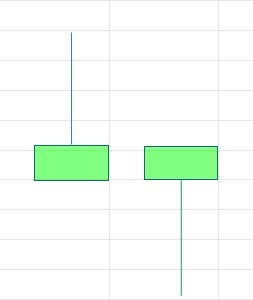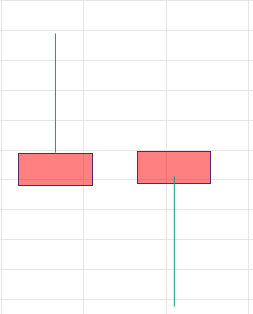# Code to find completed pin bar

Hi,

I want to prepare a pin bar detection scanner that will detect pin bars with 1 hour timeframe with following logic-

1. body of the pin bar should be less than 0.4% of the stock price

2. Wick of pin bar should be greater than 0.8%. of the stock priceLogic for above kind of pinbars is (Please remember I am talking of single candle pattern. So either hammer or inverted hammer with green body)

( (REF(OPEN,1)>REF(CLOSE,1)) AND (((REF(OPEN,1)-REF(CLOSE,1))*100)/REF(CLOSE,1)<0.4) AND ( ((((REF(HIGH,1)-REF(CLOSE,1))*100)/REF(CLOSE,1))>0.8) OR ((((REF(OPEN,1)-REF(LOW,1))*100)/REF(CLOSE,1))>0.8) ))Logic for above pin bar is  (Please remember I am talking of single candle pattern. So either hammer or inverted hammer with red body)​

((REF(CLOSE,1)>REF(OPEN,1)) AND (((REF(CLOSE,1)-REF(OPEN,1))*100)/REF(CLOSE,1)<0.4) AND ( ((((REF(HIGH,1)-REF(OPEN,1))*100)/REF(CLOSE,1))>0.8) OR ((((REF(CLOSE,1)-REF(LOW,1))*100)/REF(CLOSE,1))>0.8) ))

Combined logic I wrote in screener script is OR of above codes-

((REF(OPEN,1)>REF(CLOSE,1)) AND (((REF(OPEN,1)-REF(CLOSE,1))*100)/REF(CLOSE,1)<0.4) AND ( ((((REF(HIGH,1)-REF(CLOSE,1))*100)/REF(CLOSE,1))>0.8) OR ((((REF(OPEN,1)-REF(LOW,1))*100)/REF(CLOSE,1))>0.8) ))  OR

((REF(CLOSE,1)>REF(OPEN,1)) AND (((REF(CLOSE,1)-REF(OPEN,1))*100)/REF(CLOSE,1)<0.4) AND ( ((((REF(HIGH,1)-REF(OPEN,1))*100)/REF(CLOSE,1))>0.8) OR ((((REF(CLOSE,1)-REF(LOW,1))*100)/REF(CLOSE,1))>0.8) ))

I didn't use SET functionality because more than 1 SET is not supported in scanner.

I ran the scanner in live market with list of 60 stocks but Its not generating any signal even after all conditions of code are met.(I checked the charts and pin bars with my specification were forming). Can you please tell where is the mistake in my code?

you can check the bullish and bearish hammer code from the Expert Advisor page

Hi Zerodha,